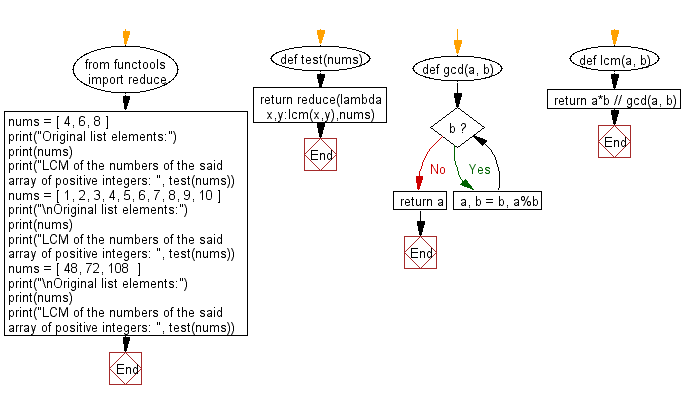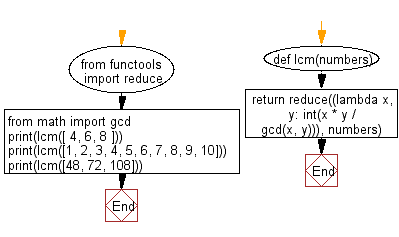﻿ Python: Least Common Multiple (LCM) of more than two numbers - w3resource# Python: Least Common Multiple (LCM) of more than two numbers

## Python Basic - 1: Exercise-135 with Solution

Write a Python program to get the Least Common Multiple (LCM) of more than two numbers. Take the numbers from a given list of positive integers.

From Wikipedia,
In arithmetic and number theory, the least common multiple, lowest common multiple, or smallest common multiple of two integers a and b, usually denoted by lcm(a, b), is the smallest positive integer that is divisible by both a and b. Since division of integers by zero is undefined, this definition has meaning only if a and b are both different from zero. However, some authors define lcm(a,0) as 0 for all a, which is the result of taking the lcm to be the least upper bound in the lattice of divisibility.

Sample Solution-1:

Python Code:

``````from functools import reduce
def test(nums):
return reduce(lambda x,y:lcm(x,y),nums)
def gcd(a, b):
while b:
a, b = b, a%b
return a
def lcm(a, b):
return a*b // gcd(a, b)
nums = [ 4, 6, 8 ]
print("Original list elements:")
print(nums)
print("LCM of the numbers of the said array of positive integers: ", test(nums))
nums = [ 1, 2, 3, 4, 5, 6, 7, 8, 9, 10 ]
print("\nOriginal list elements:")
print(nums)
print("LCM of the numbers of the said array of positive integers: ", test(nums))
nums = [ 48, 72, 108  ]
print("\nOriginal list elements:")
print(nums)
print("LCM of the numbers of the said array of positive integers: ", test(nums))
``````

Sample Output:

```Original list elements:
[4, 6, 8]
LCM of the numbers of the said array of positive integers:  24

Original list elements:
[1, 2, 3, 4, 5, 6, 7, 8, 9, 10]
LCM of the numbers of the said array of positive integers:  2520

Original list elements:
[48, 72, 108]
LCM of the numbers of the said array of positive integers:  432
```

Flowchart:## Visualize Python code execution:

The following tool visualize what the computer is doing step-by-step as it executes the said program:

Sample Solution-2:

Use functools.reduce(), math.gcd() and lcm(x,y) = x * y / gcd(x,y) over the given list.

Python Code:

``````from functools import reduce
from math import gcd

def lcm(numbers):
return reduce((lambda x, y: int(x * y / gcd(x, y))), numbers)

print(lcm([ 4, 6, 8 ]))
print(lcm([1, 2, 3, 4, 5, 6, 7, 8, 9, 10]))
print(lcm([48, 72, 108]))
``````

Sample Output:

```24
2520
432
```

Flowchart:## Visualize Python code execution:

The following tool visualize what the computer is doing step-by-step as it executes the said program:

Python Code Editor:

Have another way to solve this solution? Contribute your code (and comments) through Disqus.

What is the difficulty level of this exercise?

Test your Programming skills with w3resource's quiz.

﻿

## Python: Tips of the Day

Iterating over dictionaries using 'for' loops:

I am a bit puzzled by the following code: d = {'x': 1, 'y': 2, 'z': 3} for key in d: print key, 'corresponds to', d[key] What I don't understand is the key portion. How does Python recognize ...

key is just a variable name.

```for key in d:
```

For Python 3.x:

```for key, value in d.items():
```

For Python 2.x:

```for key, value in d.iteritems():
```

To test for yourself, change the word key to poop.

In Python 3.x, iteritems() was replaced with simply items(), which returns a set-like view backed by the dict, like iteritems() but even better. This is also available in 2.7 as viewitems().

The operation items() will work for both 2 and 3, but in 2 it will return a list of the dictionary's (key, value) pairs, which will not reflect changes to the dict that happen after the items() call. If you want the 2.x behavior in 3.x, you can call list(d.items()).

Ref: https://bit.ly/37dm0Qo